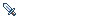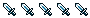Party system suggestion

Got something on your mind about the project? This is the correct place for that.
Forum rules
This forum is for feature requests, content changes additions, anything not a Bug in the software.
Please report all bugs on the Support Forums
Blamoo
PeonPosts: 98
Joined: 03 Feb 2006, 23:07

Party system suggestion

Exp sharing system for party:
a member kill something and get X exp
the average exp is calculated (Y members = (X/Y))
every member share 50% exp + member defined % (0 ~ 50)
50% + member defined value = % shared exp per member
% shared exp per member/number of members = % total shared exp
Total shared exp + saved exp = exp gained...

e.g.:
3 party members
situation:
a member killed something and got 300 exp

calculation: 3 members / 300 exp = 100 exp
member 1 define: share 30% exp + 50% (this value is the same for everyone)
member 2 define: share 0% exp +50%
member 3 define: Share 50% exp +50%

sharing:
member 1: 30% + 50% = 80% - share 80 exp keep 20 exp
member 2: 0% + 50% = 50% - share 50 exp keep 50 exp
member 3: 50% + 50% = 100% - share 100 exp keep 0 exp
total: (Exp1+exp2+exp3)/number of members = (80+50+100)/3 = 76,66...

member 1: keep 20 exp + 76,66 = 97(,66) exp
member 2: keep 50 exp + 76,66 = 126(,66) exp
member 3: keep 0 exp + 76,66 = 76(,66) exp
97 + 126 + 76 = 299 (300 exp) = total exp got

Example: http://geocities.yahoo.com.br/blamoo2/Expshare.zip
Bjørn